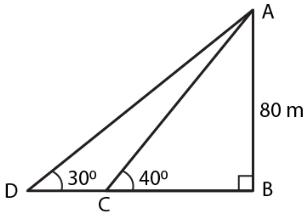Guru

# As observed from the top of a 80 m tall light house, the angles of depression of two ships on the same side of the light house in horizontal line with its base are 30 and 40 respectively. Find the distance between the two ships. Give your answer correct to the nearest metre.

• 0

sir this is the important  question from the book -ML aggarwal( avichal publication) class 10th , chapter20 , heights and distances
we have the information about the qustion that As observed from the top of a 80 m tall light house,
the angles of depression of two ships on the same side of the light house in horizontal line with its base are 30 and 40 respectively.

Find the distance between the two ships.
Give your answer correct to the nearest metre.

question no 26 , heights and distances , ICSE board

Share

1. Consider AB as the light house and C and D as the two ships.tan 300 = AB/BD

Substituting the values

1/√3 = 80/BD

So we get

BD = 80√3…… (1)

In triangle ACB

tan 400 = AB/BC

Substituting the values

0.84 = 80/BC

So we get

BC = 80/0.84 = 95.25

Using equation (1)

BD = 80 √3 = 80 × 1.73 = 138.4

Here we get

DC = BD – BC

DC = 138.4 – 95.25 = 43.15

Therefore, the distance between the two ships is 43.15 m.

• 0